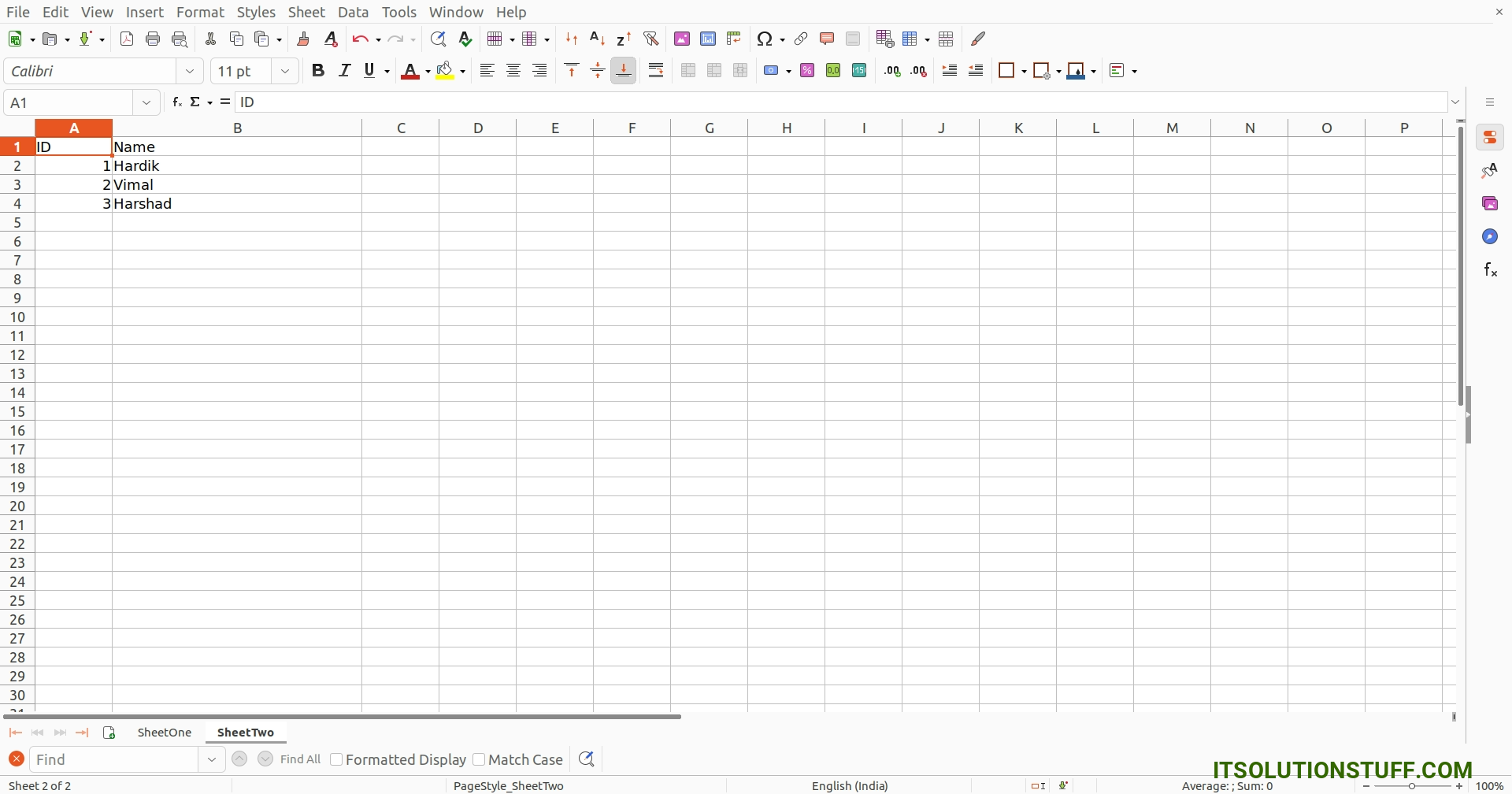# Python Read Excel File with Multiple Sheets Example

By Hardik Savani October 30, 2023 Category : Python

Hi Guys,

This tutorial is focused on python read excel file with multiple sheets. you will learn how to read multiple sheets in excel using python. step by step explain how to read data from multiple sheets in excel using python. I am going to show you about how to read excel with multiple sheets in python. Let's get started with python read excel file with multiple sheets.

There are several ways to read xlsx file with multiple sheets in python. i will give you two examples using Pandas and Openpyxl library. so let's see one by one examples.

You can use these examples with python3 (Python 3) version.

I simply created data.xlsx file with SheetOne and SheetTwo as like below, we will use same file for both example:

demo.xlsx: SheetOnedemo.xlsx: SheetTwoExample 1: using Pandas

main.py

```# import pandas lib as pd
import pandas as pd

# Read Demo file with SheetOne and SheetTwo

sheetOne = dataFrame.get('SheetOne')
sheetTwo = dataFrame.get('SheetTwo')

print(sheetOne)
print(sheetTwo)
```

Output:

```   ID             Name              Email
0   1    Hardik Savani   hardik@gmail.com
1   2  Vimal Kashiyani    vimal@gmail.com

ID     Name
0   1   Hardik
1   2    Vimal

```

Example 2: using Openpyxl

main.py

```import openpyxl

# Define variable to load the dataframe

sheetOne = dataframe['SheetOne']

# Iterate the loop to read the cell values
for row in range(0, sheetOne.max_row):
for col in sheetOne.iter_cols(1, sheetOne.max_column):
print(col[row].value)

sheetTwo = dataframe['SheetTwo']

# Iterate the loop to read the cell values
for row in range(0, sheetTwo.max_row):
for col in sheetTwo.iter_cols(1, sheetTwo.max_column):
print(col[row].value)
```

Output:

```ID
Name
Email
1
Hardik Savani
hardik@gmail.com
2
Vimal Kashiyani
vimal@gmail.com
3

ID
Name
1
Hardik
2
Vimal
3